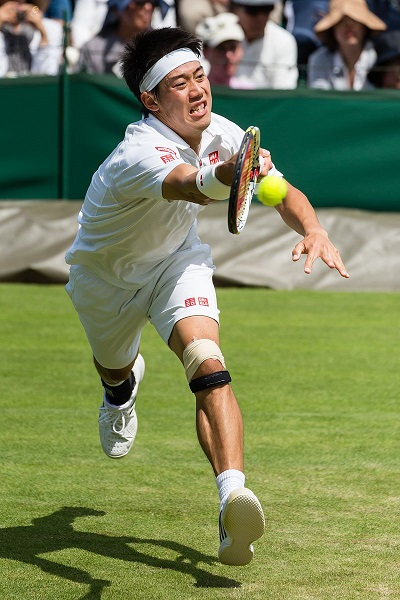﻿ Mechanics Map - Impulse and Momentum in Particle Systems

# Impulse and Momentum in Particle Systems

The concepts of Impulse and Momentum provide a third method of solving kinetics problems in dynamics. Generally this method is called the Impulse-Momentum Method, and it can be boiled down to the idea that the impulse exerted on a body over a given time will be equal to the change in that body's momentum. The impulse is usually denoted by the variable J (not to be confused with the polar area moment of inertia, which is also J) and the momentum is a body's mass times it's velocity. Impulses and velocities are both vector quantities, giving us the basic equation below.

 $\vec{J} = m \vec{v_{f}}-m \vec{v_{i}}$

Before we go into depth on using the method, it will first be important to define the key concepts of impulse and momentum.

## Impulse:

The concept of an impulse in it's most basic form is a force integrated over a time. For a force with a constant magnitude, we can find the magnitude of the impulse by multiplying the magnitude of the force by the time that force is exerted. If the force is not constant, we simply integrate the force function over the set time period. The direction of the impulse vector will be the direction of the force vector and the units will be a force times a time (Newton Seconds or Pound Seconds for example).

 Constant Magnitude Force: $\vec{J} = \vec{F}*t$ Non-Constant Magnitude Force: $\vec{J} = \int \vec{F(t)} dt$

In many cases, we will discuss impulsive forces. This is an instance where we have very large forces acting over a very short time frame. In instances of impulsive forces, it is often difficult to measure the exact magnitude of the force or the time. In these cases we may only be able to deduce the magnitude of the impulse as a whole via the observed change in momentum of the body.The force the tennis racket exerts on the ball will be very large, but it will be exerted over a very short period of time. Because of this, the force is considered an "impulsive" force. It would be difficult to determine the exact magnitude of the force or time frame of the impact, but by examining the velocity of the ball before and after the impact we could deduce the overall magnitude of the impulse as a whole. Photo by DAVID ILIFF. License: CC BY-SA 3.0

## Momentum:

The momentum of a body is equal to the mass of the body times it's current velocity. Since velocity is a vector, the momentum will also be a vector, having both magnitude and a direction. Unlike the impulse, which happens over some set period of time, the momentum is captured as a snapshot of a specific instant in time (usually right before and after some impulse is exerted). The units for momentum will be mass times unit distance per unit time. This is usually kilogram meters per second in metric, or slug feet per second in US Customary units.

Momentum: $m*\vec{v}$

## Impulse and Momentum a Vector Quantities

When working with both the impulse and momentum values, it is important to remember that they are vector quantities. Just as we have done with forces and accelerations in previous sections, the first step in many impulse momentum problems in two or three dimensions is to break the quantities into components. Only once we have the scalar impulse and momentum components in our equations can we solve for unknowns.